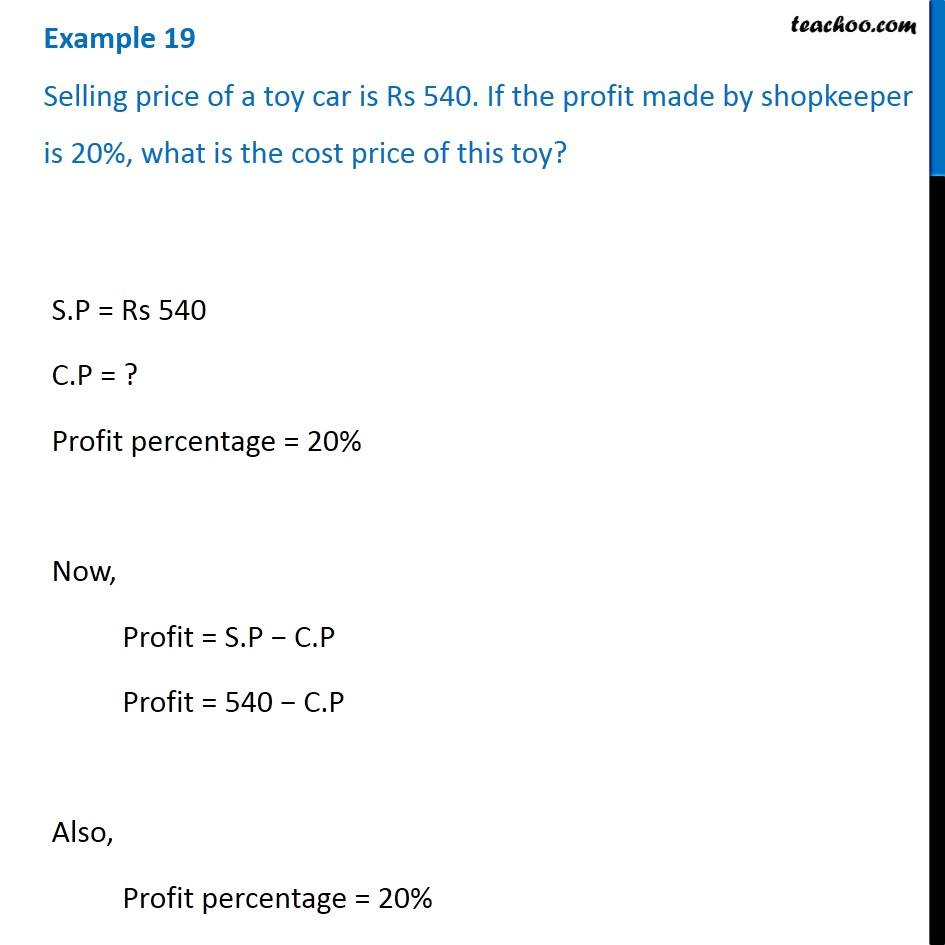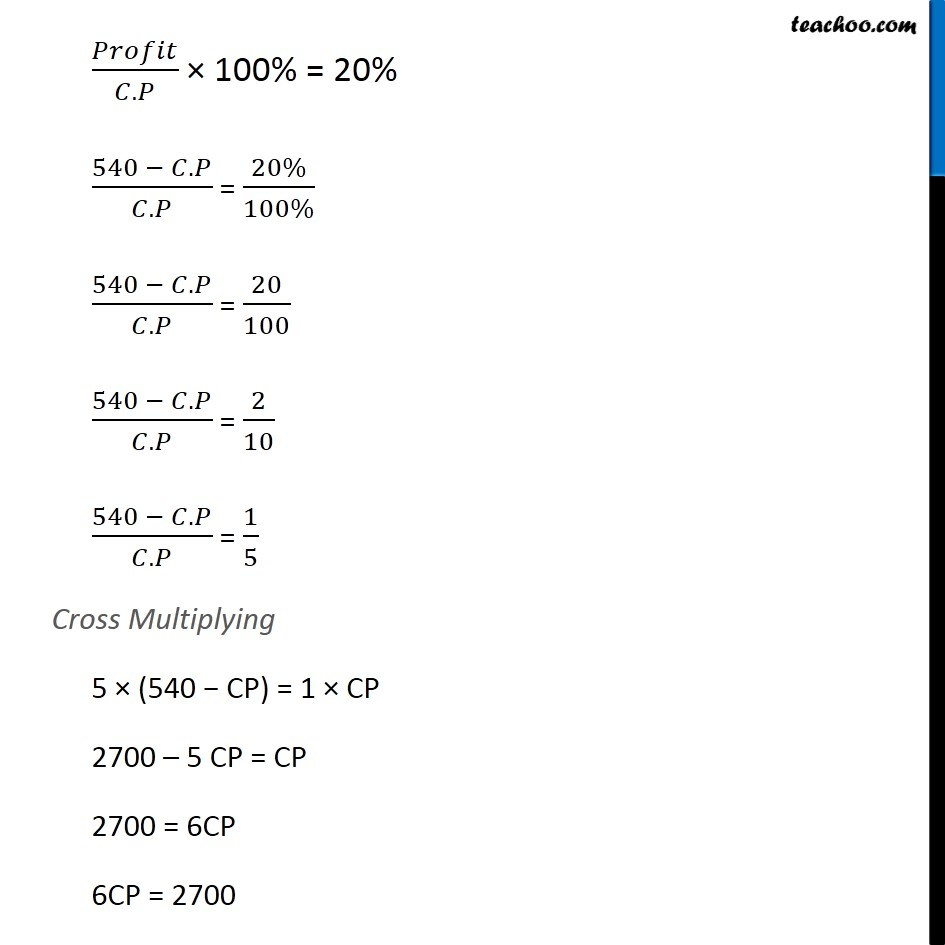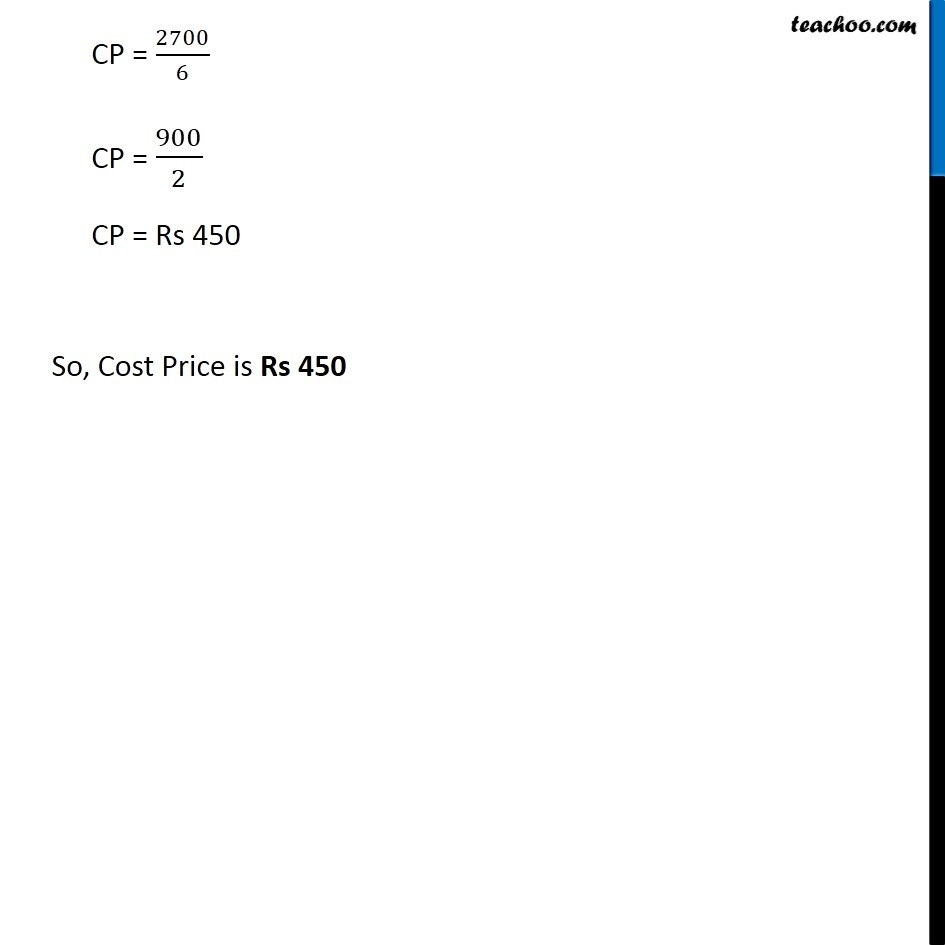Examples

Chapter 8 Class 7 Comparing Quantities
Serial order wiseGet live Maths 1-on-1 Classs - Class 6 to 12

### Transcript

Example 19 Selling price of a toy car is Rs 540. If the profit made by shopkeeper is 20%, what is the cost price of this toy? S.P = Rs 540 C.P = ? Profit percentage = 20% Now, Profit = S.P − C.P Profit = 540 − C.P Also, Profit percentage = 20% 𝑃𝑟𝑜𝑓𝑖𝑡/(𝐶.𝑃) × 100% = 20% (540 − 𝐶.𝑃)/(𝐶.𝑃) = (20%)/(100%) (540 − 𝐶.𝑃)/(𝐶.𝑃) = 20/100 (540 − 𝐶.𝑃)/(𝐶.𝑃) = 2/10 (540 − 𝐶.𝑃)/(𝐶.𝑃) = 1/5 Cross Multiplying 5 × (540 − CP) = 1 × CP 2700 – 5 CP = CP 2700 = 6CP 6CP = 2700 CP = 2700/6 CP = 900/2 CP = Rs 450 So, Cost Price is Rs 450